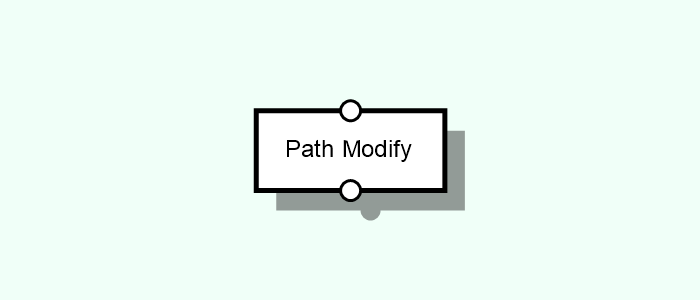# Path Modify

Applies sequences of transformations to the given input path.Tags: Path, Blend, Cleanup, Edge Cleanup, Increase Precision, Round, Simplify, Smooth, Trim

## Parameters#

Operation [Multi-Type List]
The operation to be applied to the mesh.
Blend [Compound]
Blends edges and vertices within paths. Intersecting edges result in shared vertices. Overlapping vertices are joined.
Cleanup [Compound]
Performs path cleaning by grouping/merging vertices that are closer than a specified range. This avoids possible issues when, for instance, transforming the path into a mesh.
Distance [Float]
Maximum distance between vertices allowed before a merge between them will take place. (Entity Expression reads values from individual vertices)
Cross Only [Boolean]
Indicates if the cleanup should only apply to path crossings (i.e. vertices with more than 2 edges)
Edge Cleanup [Compound]
Performs edge cleanup by merging edges that are too close together.
Width [Float]
Expected size around the edge that must be kept clean. Half of this size is used for each side of the edge. Evaluated per path edge.
Angle Tolerance [Float]
Minimum angle tolerance (in degrees) between edges in order for them to be included in the cleanup. This value should only be lowered if very small angles are known to exist, otherwise it could result in mathematical precision problems.
Increase Precision [Compound]
Increases the number of vertices in which the path is divided.
Max Segment Size [Float]
The maximum size of each one of the path segments.
Round [Compound]
Rounds the coordinate values of the path vertices, reducing possible mathematical errors in floating point values.
Precision [Int]
Number of decimal cases to which the values should be rounded to.
Simplify [Compound]
Simplifies paths by removing vertices which do not introduce a noticeable detail. Only affects vertices shared by two and only two edges.
Angle Tolerance [Float]
The angle tolerance, in degrees. Vertices whose edge angles differ less than this value from 180 degrees (meaning that they are rather straight) are removed.
Smooth [Compound]
Smooths a path by introducing more vertices following a bezier function.
Smooth Steps [Int]
The number of smoothing steps to use. The higher, the smoother it can look.
Bend [Float]
The intensity of the smoothing.
Trim [Compound]
Trims the paths leaf edges, i.e. those edges that contain non-shared vertices, by a certain amount.
Amount [Float]
The amount to cut on the leaf edges.

## Inputs#

• Input [Single | Path]: The Path to process.

## Outputs#

• Output [Path]: The Path that was processed.